# Astro Statistics: How Astro is being used

Published:

This is a collection of interesting statistics related to Astro.js. All of the data was gathered by us based on public NPM data and calculated in the most neutral way possible. The graph illustrations can be used outside this site, as long as you link us as the source.

## How many Astro sites use React25.8% of Astro.js sites use React, and 74.2% don't use React.

The React popularity calculation is based on the 76,213 downloads of `@astrojs/react`, divided by 302,931 downloads of `astro`, resulting in 25.8% usage of React. (data based on last 30 days: from 2023-01-24 to 2023-02-24)

However, Astro.js sites can utilize React in two ways: either on both build time and client side or only on build time (during SSR / SSG). There is no way for us to know which way users tend to use it, but we estimate that most people use it on the client side too.

## The popularity of Tailwind among Astro sites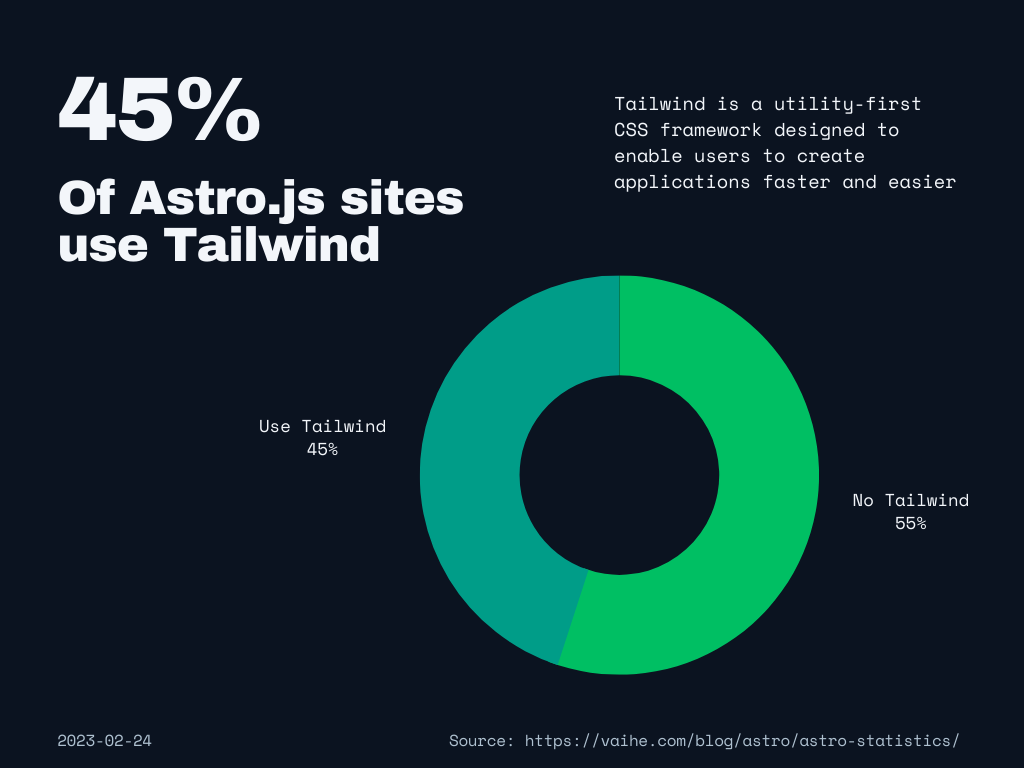45% of Astro.js sites use Tailwind, and 55% don't use tailwind.

The Tailwind statistic calculation is based on the 135,875 downloads of `@astrojs/tailwind`, divided by 302,931 downloads of `astro`, resulting in 45% usage of Tailwind. (data based on last 30 days: from 2023-01-24 to 2023-02-24)

We were very sceptical of how popular Tailwind was with Astro, so we did a small quiz among Astro users. Out of 17 people who answered a "yes or no" question on whether they use Tailwind with Astro, 8 used Tailwind, that's 47.1%. That gives at least some credibility to this statistic, but the sample size is quite small. However, one of the core members of Astro's team stepped in and mentioned that Tailwind always ranks among top 3 search results on the official Astro documentation search bar every month, which gives more credibility to this statistic.

All of this tends to make us believe that this statistic is correct and that Tailwind is a lot more popular than we had thought! One user commented that "I think the (Astro) plugin makes me use tailwind more than in other frameworks because I know I won't have to waste much time configuring stuff", which could be partly a reason for the popularity.

## Estimating how many Astro sites use SSR vs. SSG

As Astro sites that wish to use SSR require an adapter, we can estimate how many Astro.js use SSR vs. SSG by calculating the usage of supported adapters.

This calculation was done by combining the installation amounts of `@astrojs/node` (26,254), `@astrojs/vercel` (15,124), `@astrojs/cloudflare` (11,902), `@astrojs/netlify` (10,020), and `@astrojs/deno` (2,034), which came out to a total of 63,803. That total amount being divided by 302,931 downloads of `astro` results in 21% usage of SSR, which means 79% usage of SSG. (data based on last 30 days: from 2023-01-24 to 2023-02-24)

## How popular each Astro.js SSR solution is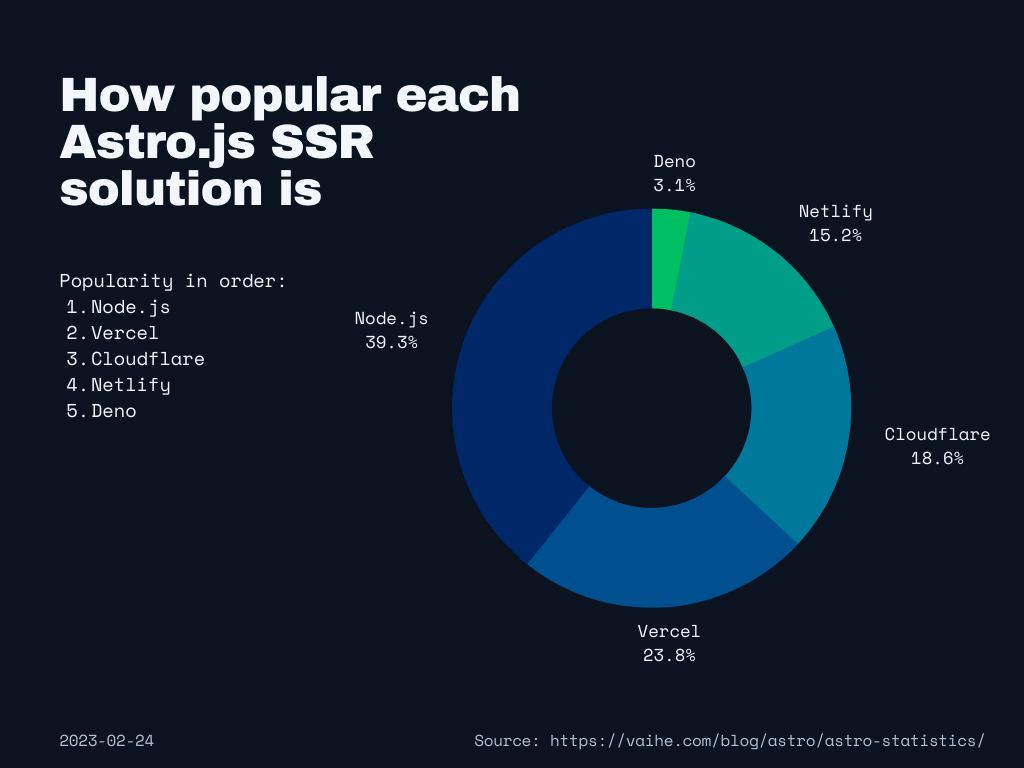The most popular SSR solutions for Astro.js are Node.js at 39.3%, Vercel at 23.8%, Cloudflare at 18.6%, Netlify at 15.2% and Deno at 3.1%.

The calculation of SSR platform and framework popularity was done by dividing each one of them by the combined installs of `@astrojs/node` (25,062), `@astrojs/vercel` (15,124), `@astrojs/cloudflare` (11,902),`@astrojs/netlify` (9,720), and `@astrojs/deno` (1,995), which came out to total of 63,803. (data based on last 30 days: from 2023-01-24 to 2023-02-24)

## How many Astro.js sites use a JavaScript framework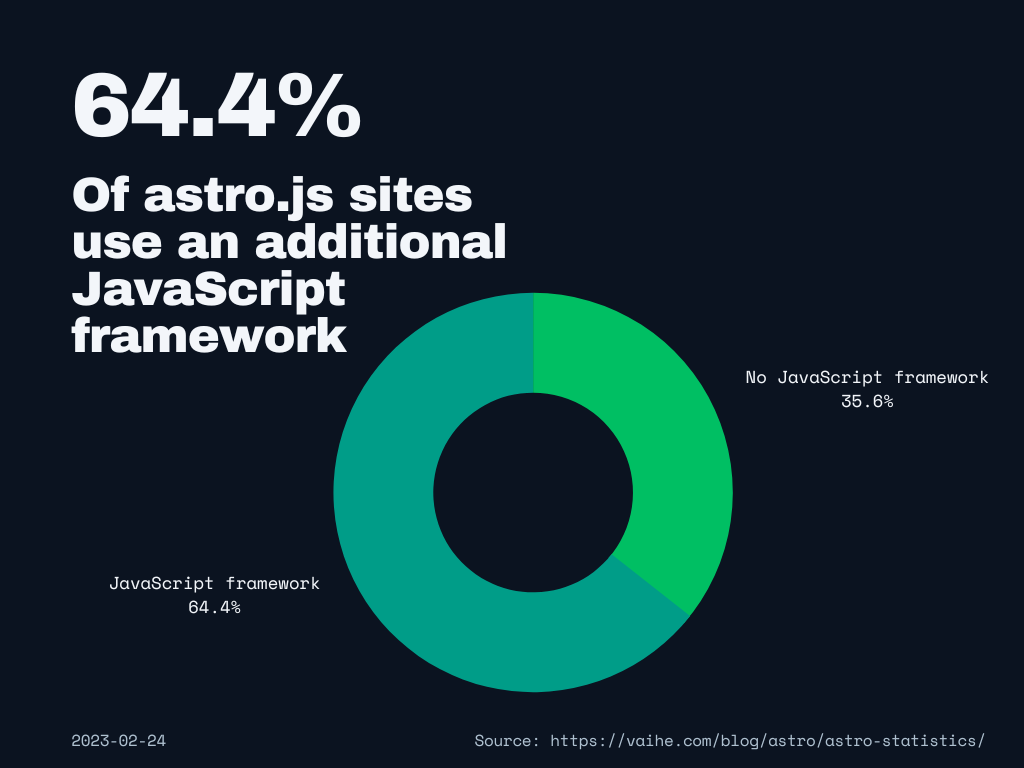64.4% of Astro.js sites use a JavaScript framework, and 35.6% of them don't.

The calculation was done by combining the total amount of downloads on `@astro/vue` (34,446), `@astrojs/lit` (6,709), `@astrojs/react` (78,825), `@astrojs/svelte` (34,348),`@astrojs/solid-js` (12,546), `@astrojs/preact` (24,774), and `@astrojs/alpinejs` (3,384). That amount (195,032) being divided by the total amount of downloads on `astro` (302,931) is 64.4%. (data based on last 30 days: from 2023-01-24 to 2023-02-24)

## The most popular JavaScript frameworks within Astro.js sites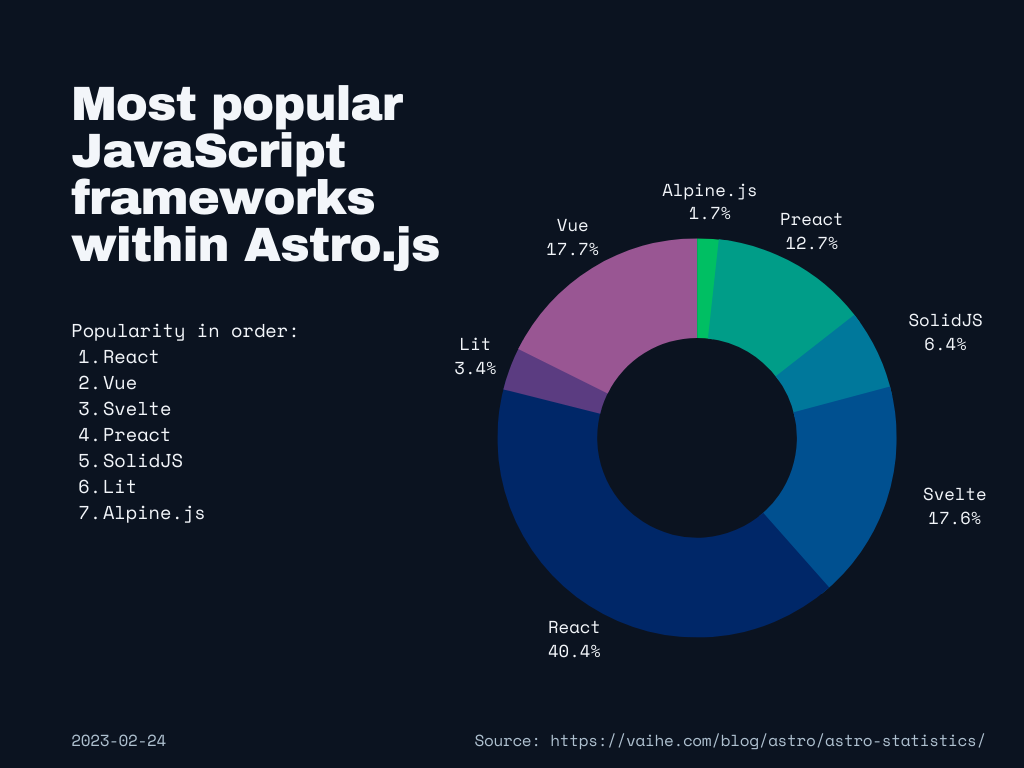Out of those Astro.js sites that use a JavaScript framework, 40.4% use React, 17.7% use Vue, 17.6% use Svelte, 12.7% use Preact, 6.4% use SolidJS, 3.4% use Lit, and 1.7% use Alpine.js.

This comparison was done by comparing the downloads of `@astro/vue` (34,446), `@astrojs/lit` (6,709), `@astrojs/react` (78,825), `@astrojs/svelte` (34,348),`@astrojs/solid-js` (12,546), `@astrojs/preact` (24,774), and `@astrojs/alpinejs` (3,384). (data based on last 30 days: from 2023-01-24 to 2023-02-24)

## The popularity of Astro vs. Gatsby vs. Next.js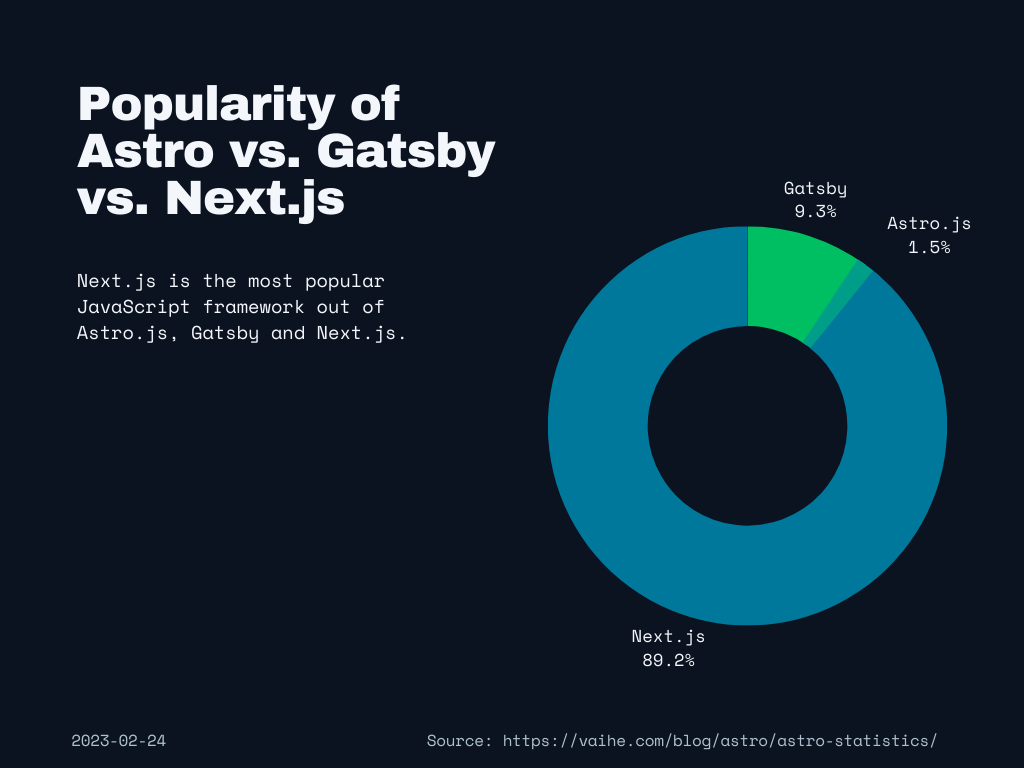When comparing the popularity of Next.js, Gatsby, and Astro.js, the first place is taken by Next.js at 89.2%, the second is Gatsby at 9.3%, and third is Astro.s at 1.5%.

This statistic was calculated by comparing each of the NPM installation counts. `Next.js` at 17,772,110, `Gatsby` at 1,855,791, and `Astro.js` at 302,931. (data based on last 30 days: from 2023-01-24 to 2023-02-24)

## MDX popularity among Astro.js sites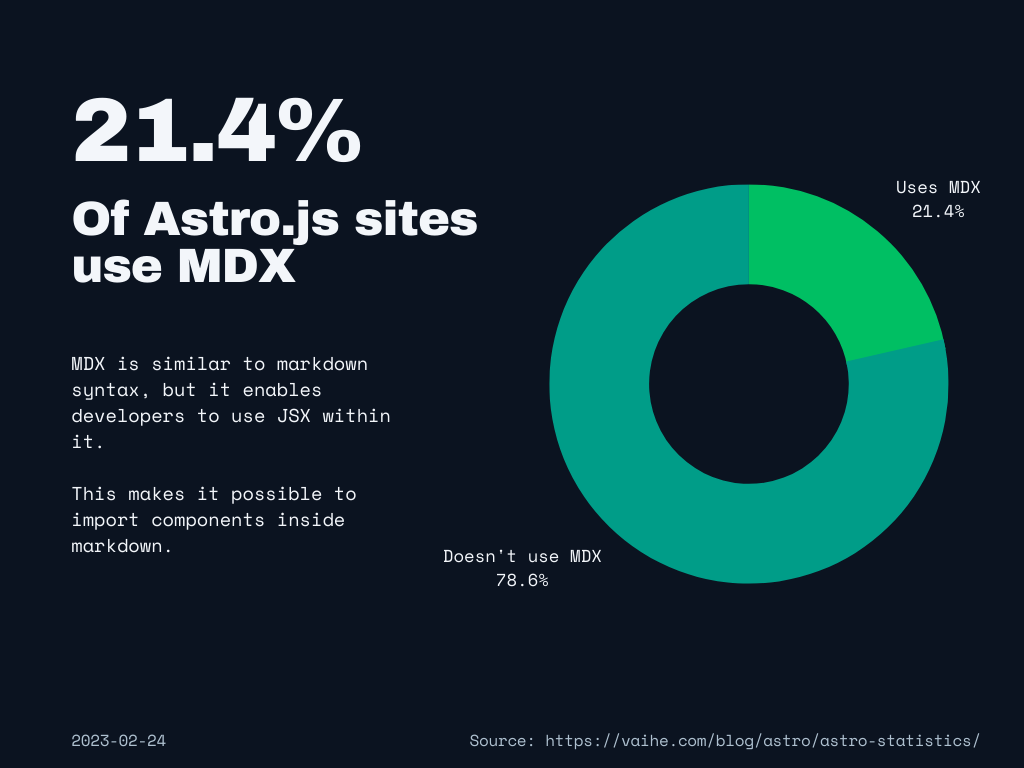21.4% of Astro.js sites use MDX, and 78.6% don't use it.

The popularity of MDX among Astro users tends to make us believe that a lot of Astro.js sites have blogs or documentation, which makes sense as Astro is a great framework for that use-case.

This statistic was calculated by dividing sites using `@astrojs/mdx` (64,938) by the total amount of `astro` installations (302,931). (data based on last 30 days: from 2023-01-24 to 2023-02-24)

## Vue popularity among Astro.js sites11% of Astro.js sites use Vue, and 89% don't use Vue.

This statistic was calculated by dividing sites using `@astrojs/vue` (33,331) by the total amount of `astro` installations (302,931). (data based on last 30 days: from 2023-01-24 to 2023-02-24)